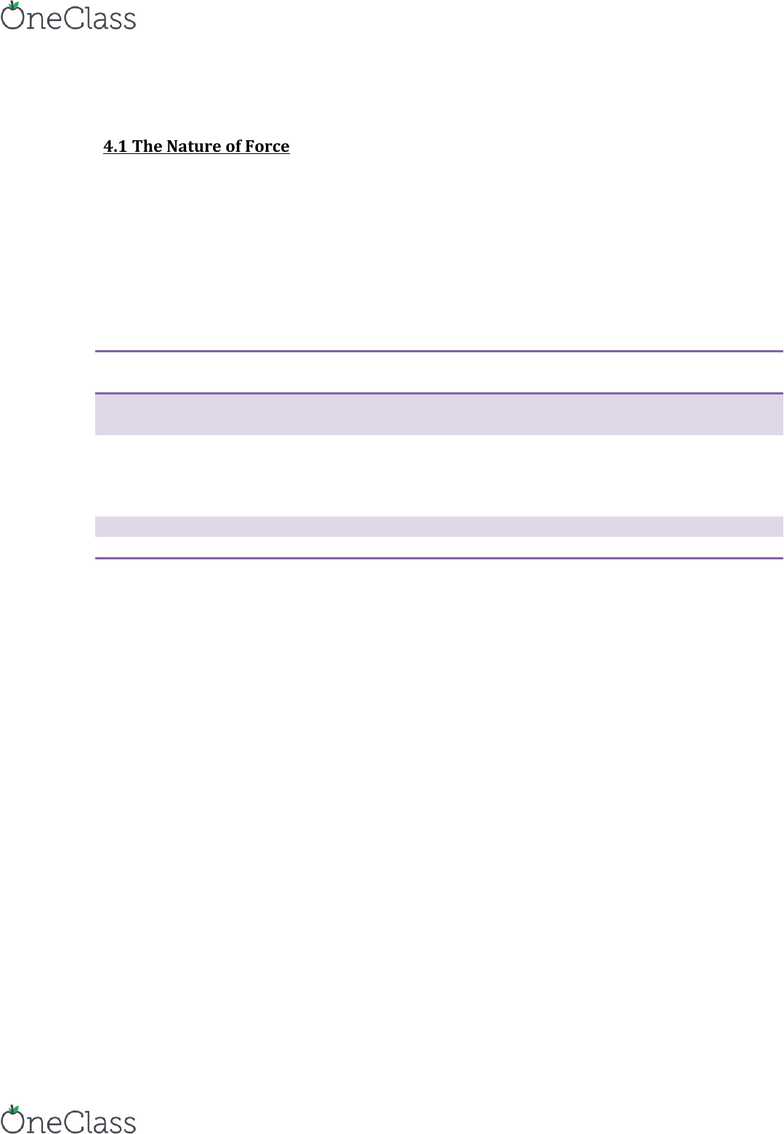Study Guides (390,000)
CA (150,000)
McMaster (9,000)
PHYSICS (100)
Midterm

# PHYSICS 1L03 Study Guide - Midterm Guide: Net Force, Flowchart

Department
Physics
Course Code
PHYSICS 1L03
Professor
Christine Wilson
Study Guide
Midterm

Page:
of 6Physics Chapter IV (1) Unit II
Physics Review Chapter 4
4.1 The Nature of Force
Forces
Are all around us
Help keep our atoms and molecules together
Allow us to interact with our environment
Fundamental Forces
Force Relative
Strength
Range Function
Strong 1 Less than 10-15m Holds protons and neutrons
together
Electromagnetic Infinite Holds atoms and molecules
together causes atoms and
molecules to repel each other if
they get too close
Weak 10-5 Less than 10-18m Involved in radioactive decay
Gravitational 6 Infinite Mutual attraction of matter
Gravity is the weakest of the four forces, but it is very significant because:
It has an infinite range
It always attracts
Force is a Vector Quantity
Any force acting on an object can change the shape and/or velocity of the object.
find more resources at oneclass.com
find more resources at oneclass.com
Physics Chapter IV (2) Unit II
Symbol for force:
SI unit for force: the newton [symbol: N]
One newton is equal to one kilogram-metre per second squared [1kg∙m/s2]
The force required to move a 1-kg object with an acceleration of 1m/s2
The direction of a force is described using reference coordinates
E.g.
Representing Forces Using Free-Body Diagrams
Only the force vectors exerted on the object
Vectors are drawn with their tails meeting at the approximate centre of the object